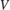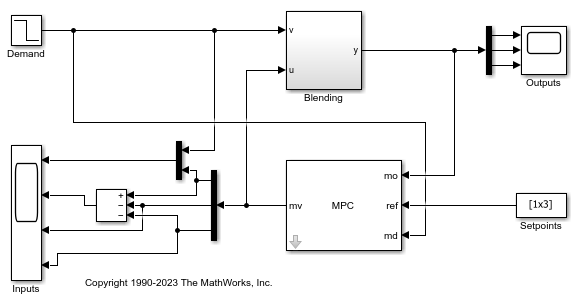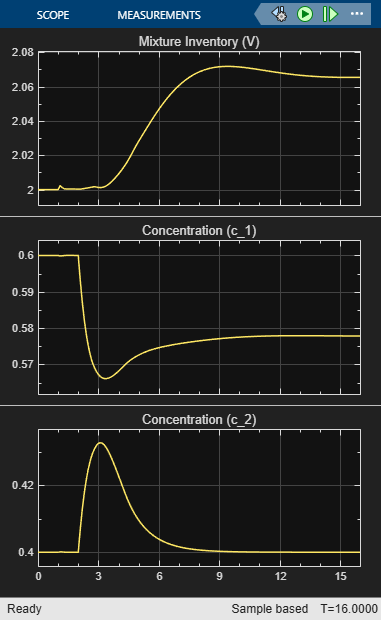# Use Custom Constraints in Blending Process

This example shows how to design an MPC controller for a blending process using custom mixed input/output constraints.

### Blending Process

A continuous blending process combines three feeds in a well-mixed container to produce a blend having desired properties. The dimensionless governing equations are:where

•is the mixture inventory (in the container).

•is the plow rate for feed.

•is the rate at which the blend is being removed from inventory, that is the demand.

•is the concentration of constituentin feed.

•is the concentration of constituentin the blend.

•is time.

In this example, there are two important constituents,= 1 and 2.

The control objectives are targets for the two constituent concentrations in the blend and the mixture inventory. The challenge is that the demand,, and feed compositions,, vary. The inventory, blend compositions, and demand are measured, but the feed compositions are unmeasured.

At the nominal operating condition:

• Feed 1,, (mostly constituent 1) is 80% of the total inflow.

• Feed 2,, (mostly constituent 2) is 20%.

• Feed 3,, (pure constituent 1) is not used.

The process design allows manipulation of the total feed entering the mixing chamber,, and the individual rates of feeds 2 and 3. In other words, the rate of feed 1 is:Each feed has limited availability:The equations are normalized such that, at the nominal steady state, the mean residence time in the mixing container is.

The constraintis imposed by an upstream process, and the constraintsare imposed by physical limits.

### Define Linear Plant Model

The blending process is mildly nonlinear, however you can derive a linear model at the nominal steady state. This approach is quite accurate unless the unmeasured feed compositions change. If the change is sufficiently large, the steady-state gains of the nonlinear process change sign, and the closed-loop system can become unstable.

Specify the number of feeds, ni, and the number of constituents, nc.

ni = 3; nc = 2; 

Specify the nominal flow rates for the three input streams and the output stream, or demand. At the nominal operating condition, the output flow rate is equal to the sum of the input flow rates.

Fin_nom = [1.6,0.4,0]; F_nom = sum(Fin_nom); 

Define the nominal constituent compositions for the input feeds, where cin_nom(i,j) represents the composition of constituent i in feed j.

cin_nom = [0.7 0.2 0.8;0.3 0.8 0]; 

Define the nominal constituent compositions in the output feed.

cout_nom = cin_nom*Fin_nom'/F_nom; 

Normalize the linear model such that the target demand is 1 and the product composition is 1.

fin_nom = Fin_nom/F_nom; gij = [cin_nom(1,:)/cout_nom(1); cin_nom(2,:)/cout_nom(2)]; 

Create a state-space model with feed flows F1, F2, and F3 as MVs:

A = [zeros(1,nc+1); zeros(nc,1) -eye(nc)]; Bu = [ones(1,ni); gij-1]; 

Change the MV definition to [FT, F2, F3] where F1 = FT - F2 - F3

Bu = [Bu(:,1), Bu(:,2)-Bu(:,1), Bu(:,3)-Bu(:,1)]; 

Add the measured disturbance, blend demand, as the 4th model input.

Bv = [-1; zeros(nc,1)]; B = [Bu Bv]; 

Define all of the states as measurable. The states consist of the mixture inventory and the constituent concentrations.

C = eye(nc+1); 

Specify that there is no direct feed-through from the inputs to the outputs.

D = zeros(nc+1,ni+1); 

Construct the linear plant model.

Model = ss(A,B,C,D); Model.InputName = {'F_T','F_2','F_3','F'}; Model.InputGroup.MV = 1:3; Model.InputGroup.MD = 4; Model.OutputName = {'V','c_1','c_2'}; 

### Create MPC Controller

Specify the sample time, prediction horizon, and control horizon for the controller.

Ts = 0.1; p = 10; m = 3; 

Create the controller.

mpcobj = mpc(Model,Ts,p,m); 
-->The "Weights.ManipulatedVariables" property of "mpc" object is empty. Assuming default 0.00000. -->The "Weights.ManipulatedVariablesRate" property of "mpc" object is empty. Assuming default 0.10000. -->The "Weights.OutputVariables" property of "mpc" object is empty. Assuming default 1.00000. 

The outputs are the inventory, y(1), and the constituent concentrations, y(2) and y(3). Specify nominal values of unity after normalization for all outputs.

mpcobj.Model.Nominal.Y = [1 1 1]; 

Specify the normalized nominal values for the manipulated variables, u(1), u(2) and u(3), and the measured disturbance, u(4).

mpcobj.Model.Nominal.U = [1 fin_nom(2) fin_nom(3) 1]; 

Specify output tuning weights. To pay more attention to controlling the inventory and the composition of the first constituent, use larger weights for the first two outputs.

mpcobj.Weights.OV = [1 1 0.5]; 

Specify the hard bounds (physical limits) on the manipulated variables.

umin = [0 0 0]; umax = [2 0.6 0.6]; for i = 1:3 mpcobj.MV(i).Min = umin(i); mpcobj.MV(i).Max = umax(i); mpcobj.MV(i).RateMin = -0.1; mpcobj.MV(i).RateMax = 0.1; end 

The total feed rate and the rates of feed 2 and feed 3 have upper bounds. Feed 1 also has an upper bound, determined by the upstream unit supplying it.

### Specify Mixed Constraints

Given the specified upper bounds on the feed 2 and 3 rates (0.6), it is possible that their sum could be as much as 1.2. Since the nominal total feed rate is 1.0, the controller can request a physically impossible condition, where the sum of feeds 2 and 3 exceeds the total feed rate, which implies a negative feed 1 rate.

The following constraint prevents the controller from requesting an unrealisticvalue.Specify this constraint in the form.

E = [-1 1 1; 1 -1 -1]; g = [0;0.8]; 

Since no outputs are specified in the mixed constraints, set their coefficients to zero.

F = zeros(2,3); 

Specify that both constraints are hard (ECR = 0).

v = zeros(2,1); 

Specify zero coefficients for the measured disturbance.

h = zeros(2,1); 

Set the custom constraints in the MPC controller.

setconstraint(mpcobj,E,F,g,v,h) 

The Simulink model contains a nonlinear model of the blending process and an unmeasured disturbance in the constituent 1 feed composition.

The Demand,, is modeled as a measured disturbance. The operator can vary the demand value, and the resulting signal goes to both the process and the controller.

The model simulates the following scenario:

• At, the process is operating at steady state.

• At, the Total Demand decreases fromto.

• At, there is a large step increase in the concentration of constituent 1 in feed 1, from 1.17 to 2.17.

Open and simulate the Simulink model.

mdl = 'mpc_blendingprocess'; open_system(mdl) sim(mdl) 
-->Converting model to discrete time. Assuming no disturbance added to measured output channel #1. -->Assuming output disturbance added to measured output channel #2 is integrated white noise. -->Assuming output disturbance added to measured output channel #3 is integrated white noise. -->The "Model.Noise" property of the "mpc" object is empty. Assuming white noise on each measured output channel.In the simulation:

• At time 0, the plant operates steadily at the nominal conditions.

• At time 1, the demand decreases by 10%, and the controller maintains the inventory close to its setpoint.

• At time 2, there is a large unmeasured increase in the concentration of constituent 1 contained in feed 1. This disturbance causes a prediction error and a large disturbance in the blend composition.

The disturbance is a nonlinear effect, but the linear MPC controller recovers well and drives the blend composition back to its setpoint

### Verify Effect of Custom Constraints

Plot the feed rate signals.

figure plot(MVs.time,[MVs.signals(1).values(:,2), ... (MVs.signals(2).values + MVs.signals(3).values), ... (MVs.signals(1).values(:,2)-MVs.signals(2).values-MVs.signals(3).values)]) grid legend('FT','F2+F3','F1')The unmeasured disturbance occurs at time 2, which requires the controller to decrease F1. During the transient, F1 becomes zero. If the mixed input/output constraint had not been included, F1 would have been negative. The controller requests for FT, F2, and F3 would have been impossible to satisfy, which would lead to a performance degradation. With the constraint included, the controller does its best given the physical limits of the system.

bdclose(mdl)